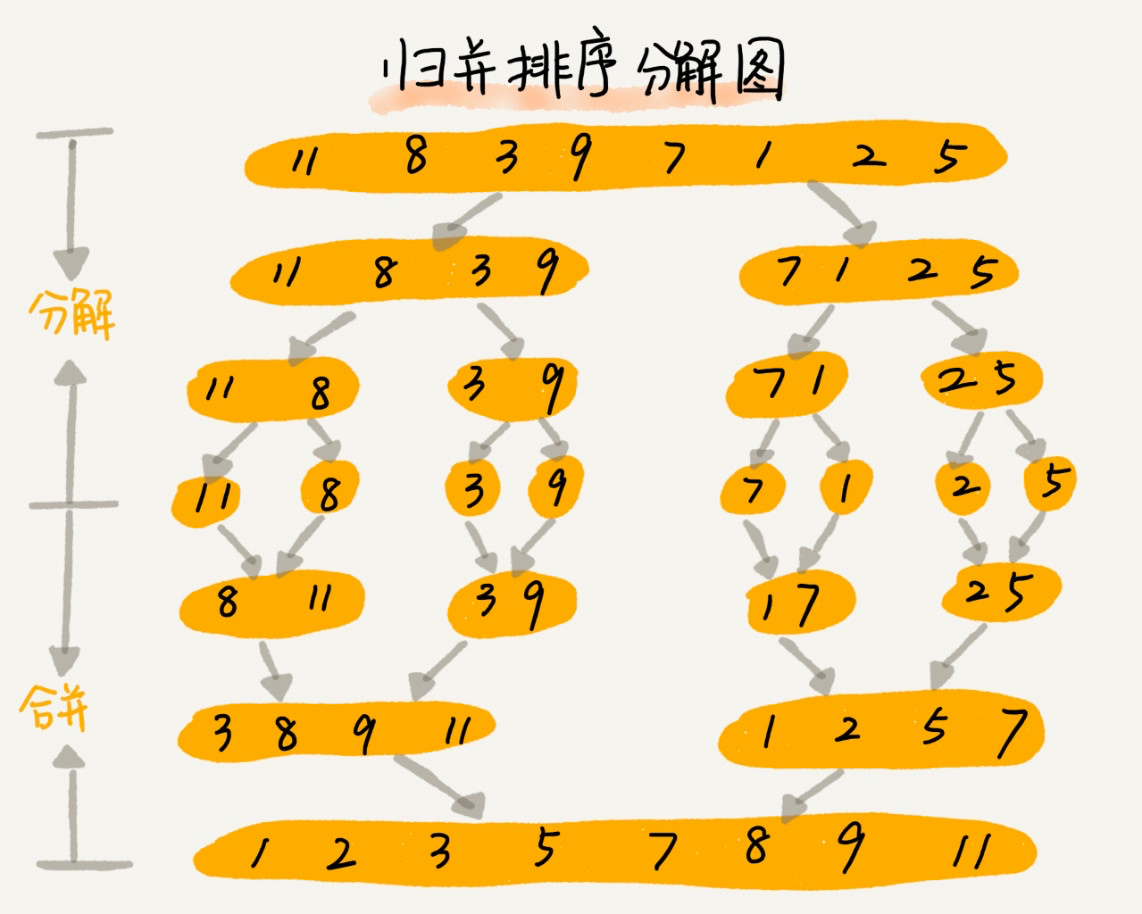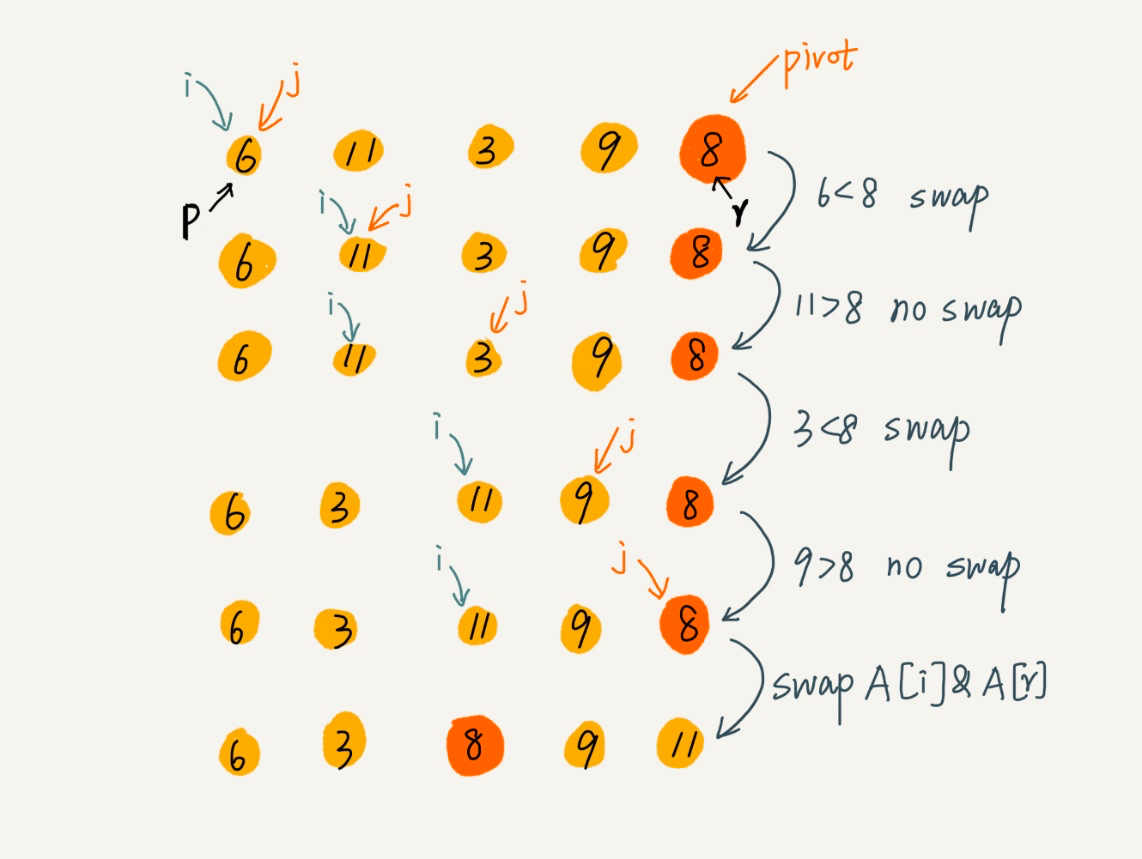# 归并排序与快速排序以及 PHP 实现### 一、分治思想

1. 分治思想：分治，顾明思意，就是分而治之，将一个大问题分解成小的子问题来解决，小的子问题解决了，大问题也就解决了。

2. 分治与递归的区别：分治算法一般都用递归来实现的。分治是一种解决问题的处理思想，递归是一种编程技巧。

### 二、归并排序#### 性能分析

1. 算法稳定性：

1. 时间复杂度：分析归并排序的时间复杂度就是分析递归代码的时间复杂度

		T(1) = C； #n=1 时，只需要常量级的执行时间，所以表示为 C。    T(n) = 2*T(n/2) + n； #n>1，其中n就是merge()函数合并两个子数组的的时间复杂度O(n)。    T(n) = 2*T(n/2) + n         = 2(2T(n/4) + n/2) + n = 4T(n/4) + 2n         = 4(2T(n/8) + n/4) + 2n = 8T(n/8) + 3*n         = 8(2T(n/16) + n/8) + 3n = 16T(n/16) + 4*n         ......         = 2^k  T(n/2^k) + k  n         ......

T(n/2^k)=T(1) 时，也就是 n/2^k=1，我们得到 k=log2n。将 k 带入上面的公式就得到T(n)=Cn+nlog2n。如用大 O 表示法，T(n)就等于O(nlogn)。所以，归并排序的是复杂度时间复杂度就是O(nlogn)

1. 空间复杂度：归并排序算法不是原地排序算法，空间复杂度是 O(n)

#### 实现

    /**     * 归并排序     * @param array $a */ public function mergeSort(array$array)    {        $low = 0;$n = count($array);$this->mergeSortRecursive($array,$low, $n - 1); } private function mergeSortRecursive(array$array, $low,$high)    {        // 递归终止条件        if ($low >=$high) {            return [$array[$high]];        }        // 取中间位置$mid$mid = $low + (($high - $low) >> 1); // 递归，合并 return$this->merge($this->mergeSortRecursive($array, $low,$mid), $this->mergeSortRecursive($array, $mid + 1,$high));    }    private function merge(array $leftArr, array$rightArr)    {        // 归并排序中最有意思的部分：可以延伸考多个有序数组合并问题        $newArr = [];$leftIndex = $rightIndex = 0;$leftCount = count($leftArr);$rightCount = count($rightArr); while ($leftIndex < $leftCount &&$rightIndex < $rightCount) {$newArr[] = $leftArr[$leftIndex] <= $rightArr[$rightIndex] ? $leftArr[$leftIndex++] : $rightArr[$rightIndex++];        }        // 这里要考虑，如果拼接完成后，数组还有剩余的处理        $start =$leftIndex;        $end =$leftCount;        $corpArr =$leftArr;        if ($rightIndex <$rightCount) {            $start =$rightIndex;            $end =$rightCount;            $corpArr =$rightArr;        }        while ($start <$end) {            $newArr[] =$corpArr[$start++]; } return$newArr;    }

### 三、快速排序

#### 性能分析

1. 算法稳定性：

1. 时间复杂度：最好、最坏、平均情况

T(1) = C； n=1 时，只需要常量级的执行时间，所以表示为 C。

T(n) = 2*T(n/2) + n； n>1

1. 空间复杂度：快排是一种原地排序算法，空间复杂度是 O(1)

#### 原地交换的实现图解#### 实现

   /**     * 快排     * @param array $array * @return array */ public function quickSort(array$array)    {        $count = count($array);        $this->quickSortRecursive($array, 0, $count - 1); return$array;    }    private function quickSortRecursive(array &$array, int$left, int $right) { if ($left >= $right) { return; }$q = $this->partition($array, $left,$right);        $this->quickSortRecursive($array, $left,$q - 1);        $this->quickSortRecursive($array, $q + 1,$right);    }    private function partition(array &$array, int$left, int $right) {$pivot = $array[$right];        $index =$left;        for ($j =$left; $j <$right; ++$j) { if ($array[$j] <$pivot) {                $tmp =$array[$j];$array[$j] =$array[$index];$array[$index] =$tmp;                $index ++; } }$tmp = $array[$index];        $array[$index] = $array[$right];        $array[$right] = $tmp; return$index;    }

### 四、归并排序与快速排序的区别

1. 归并排序，是先递归调用，再进行合并，合并的时候进行数据的交换。所以它是自下而上的排序方式。何为自下而上？就是先解决子问题，再解决父问题。

2. 快速排序，是先分区，在递归调用，分区的时候进行数据的交换。所以它是自上而下的排序方式。何为自上而下？就是先解决父问题，再解决子问题。## 评论